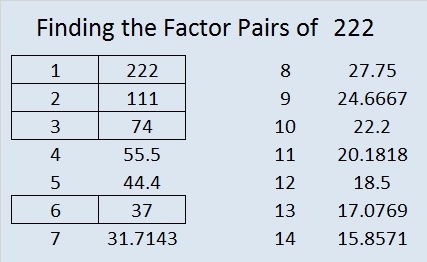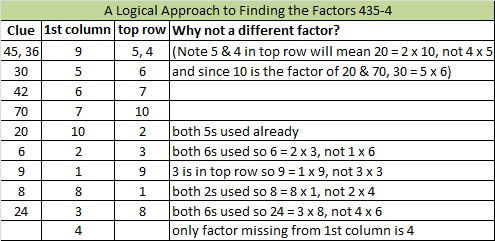# 222 and Level 4

• 222 is a composite number.
• Prime factorization: 222 = 2 x 3 x 37
• The exponents in the prime factorization are 1, 1, and 1. Adding one to each and multiplying we get (1 + 1)(1 + 1)(1 + 1) = 2 x 2 x 2 = 8. Therefore 222 has 8 factors.
• Factors of 222: 1, 2, 3, 6, 37, 74, 111, 222
• Factor pairs: 222 = 1 x 222, 2 x 111, 3 x 74, or 6 x 37
• 222 has no square factors so its square root cannot be simplified. √222 ≈ 14.8997Excel file of puzzles and previous week’s factor solutions: 10 Factors 2014-09-01